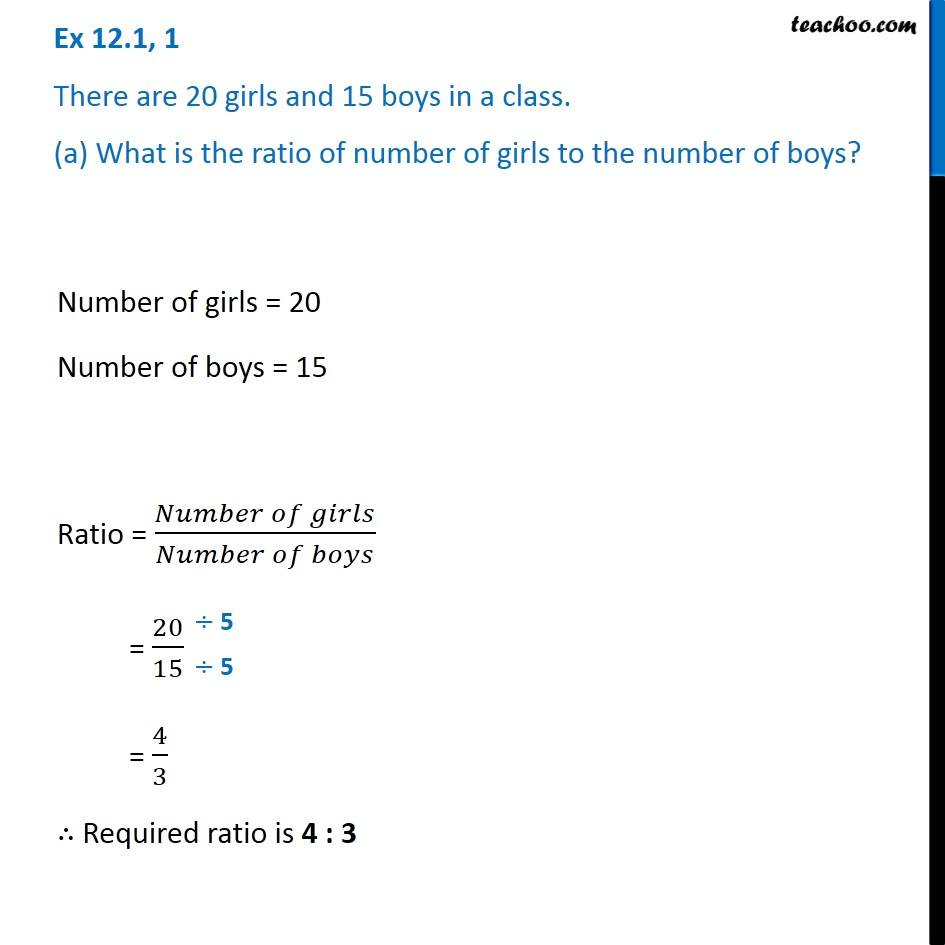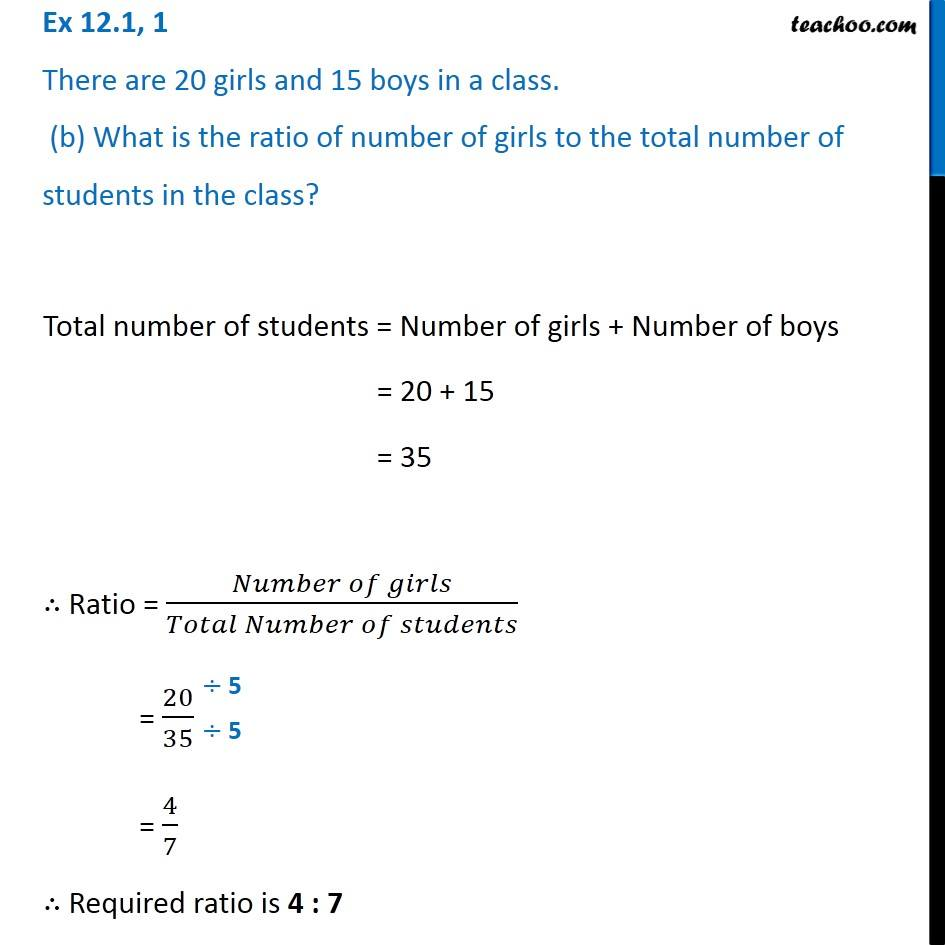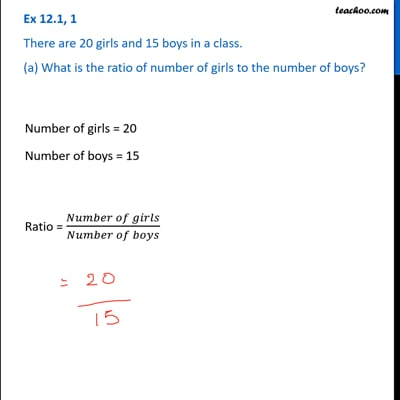Ratios

Chapter 12 Class 6 Ratio and Proportion
Concept wiseThis video is only available for Teachoo black users

Learn in your speed, with individual attention - Teachoo Maths 1-on-1 Class

### Transcript

Ex 12.1, 1 There are 20 girls and 15 boys in a class. (a) What is the ratio of number of girls to the number of boys? Number of girls = 20 Number of boys = 15 Ratio = (𝑁𝑢𝑚𝑏𝑒𝑟 𝑜𝑓 𝑔𝑖𝑟𝑙𝑠)/(𝑁𝑢𝑚𝑏𝑒𝑟 𝑜𝑓 𝑏𝑜𝑦𝑠) = 20/15 = 4/3 ∴ Required ratio is 4 : 3 Ex 12.1, 1 There are 20 girls and 15 boys in a class. (b) What is the ratio of number of girls to the total number of students in the class?Total number of students = Number of girls + Number of boys = 20 + 15 = 35 ∴ Ratio = (𝑁𝑢𝑚𝑏𝑒𝑟 𝑜𝑓 𝑔𝑖𝑟𝑙𝑠)/(𝑇𝑜𝑡𝑎𝑙 𝑁𝑢𝑚𝑏𝑒𝑟 𝑜𝑓 𝑠𝑡𝑢𝑑𝑒𝑛𝑡𝑠) = 20/35 = 4/7 ∴ Required ratio is 4 : 7